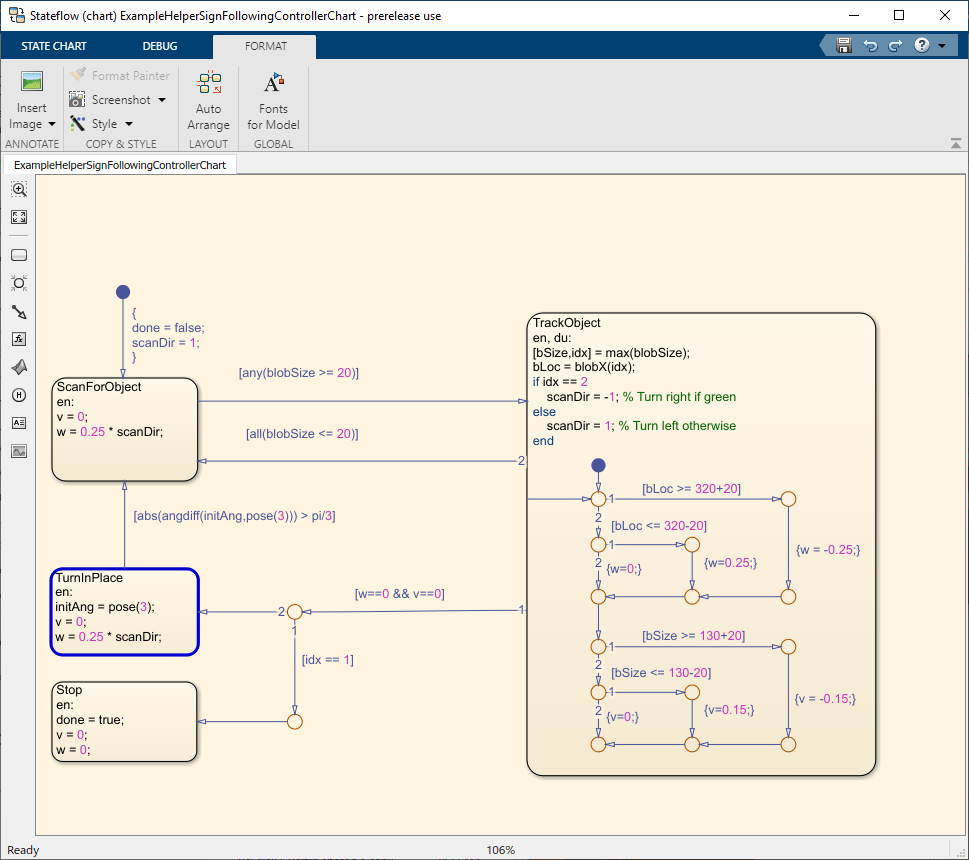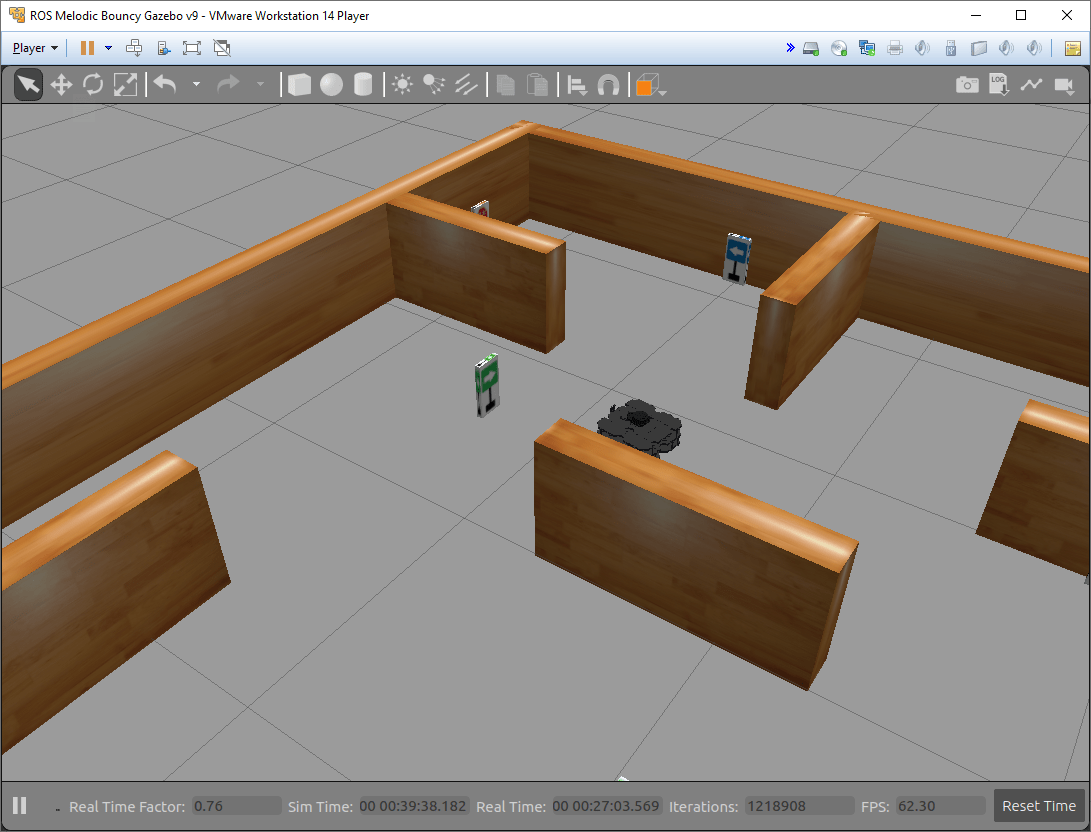## Sign Following Robot Using ROS

This example shows you how to use a ROS network to control a simulated robot running on a separate ROS-based simulator. Depending on your system, this example is provided for ROS and ROS 2 networks using either MATLAB® or Simulink®. The example shown on this page uses ROS and MATLAB. For the others, see:

### Sign Following Robot with ROS in MATLAB

This example shows you how to use MATLAB® to control a simulated robot running on a separate ROS-based simulator over a ROS network.

In this example, you run MATLAB script that implements a sign-following algorithm and controls the simulated robot to follow a path based on signs in the environment. The algorithm receives the location information and camera information from the simulated robot, which is running in a separate ROS-based simulator. The algorithm detects the color of the sign and sends the velocity commands to turn the robot based on the color. In this example, the algorithm is designed to turn left when robot encounters a blue sign and turn right when robot encounters a green sign. FInally the robot stops when it encounters a red sign.

Connect to a Robot Simulator

Start a ROS-based simulator for a differential-drive robot and configure MATLAB® connection with the robot simulator.

This example uses a virtual machine (VM) available for download at Virtual Machine with ROS 2 Melodic and Gazebo.

• Start the Ubuntu® virtual machine desktop.

• In the Ubuntu desktop, click the Gazebo Sign Follower ROS icon to start the Gazebo world built for this example.

• Specify the IP address and port number of the ROS master in Gazebo so that MATLAB® can communicate with the robot simulator. For this example, the ROS master in Gazebo is `http://192.168.203.128:11311 `and your host computer address is `192.168.31.1`.

• Start the ROS 1 network using `rosinit`.

```masterIP = '192.168.203.128'; setenv('ROS_IP','192.168.31.1'); setenv('ROS_MASTER_URI',['http://' masterIP ':11311']); rosinit(masterIP,11311)```
```The value of the ROS_IP environment variable, 192.168.31.1, will be used to set the advertised address for the ROS node. Initializing global node /matlab_global_node_02315 with NodeURI http://192.168.31.1:49592/ ```

Setup ROS Communication

Create publishers and subscribers to relay messages to and from the robot simulator over ROS network. You need subscribers for the image and odometry data. To control the robot, set up a publisher to send velocity commands using `/cmd_vel`.

```imgSub = rossubscriber("/camera/rgb/image_raw"); odomSub = rossubscriber("/odom"); [velPub, velMsg] = rospublisher("/cmd_vel", "geometry_msgs/Twist");```

Define the image processing color threshold parameters. Each row defines the threshold values for the different colors.

```colorThresholds = [100 255 0 55 0 50; ... % Red 0 50 50 255 0 50; ... % Green 0 40 0 55 50 255]'; % Blue```

Create Sign Following Controller Using Stateflow® Chart

This example provides an example helper MATLAB Stateflow® chart that takes in the image size, coordinates from processed image, and the robot odometry poses. The chart provides linear and angular velocity to drive the robot based on these inputs.

```controller = ExampleHelperSignFollowingControllerChart; open('ExampleHelperSignFollowingControllerChart');```Run Control Loop

This section runs the controller to receive images and move the robot to follow the sign. The controller does the following steps:

• Gets the latest image and odometry message from the ROS network.

• Runs the algoritm for detecting image features (`ExampleHelperSignFollowingProcessImg`).

• Generates control commands from the Stateflow® chart using `step`.

• Publishes the velocity control commands to the ROS network.

To visualize the masked image the robot sees, change the value of `doVisualization` variable to `true`.

```ExampleHelperSignFollowingSetupPreferences; % Control the visualization of the mask doVisualization = false; r = rateControl(10); receive(imgSub); % Wait to receive an image message before starting the loop receive(odomSub); while(~controller.done) % Get latest sensor messages and process them imgMsg = imgSub.LatestMessage; odomMsg = odomSub.LatestMessage; [img,pose] = ExampleHelperSignFollowingROSProcessMsg(imgMsg, odomMsg); % Run vision and control functions [mask,blobSize,blobX] = ExampleHelperSignFollowingProcessImg(img, colorThresholds); step(controller,'blobSize',blobSize,'blobX',blobX,'pose',pose); v = controller.v; w = controller.w; % Publish velocity commands velMsg.Linear.X = v; velMsg.Angular.Z = w; send(velPub,velMsg); % Optionally visualize % NOTE: Visualizing data will slow down the execution loop. % If you have Computer Vision Toolbox, we recommend using % vision.DeployableVideoPlayer instead of imshow. if doVisualization imshow(mask); title(['Linear Vel: ' num2str(v) ' Angular Vel: ' num2str(w)]); drawnow('limitrate'); end % Pace the execution loop. waitfor(r); end```

You should see the robot moving in the ROS-based robot simulator as shown below.##### SupportGet trial now